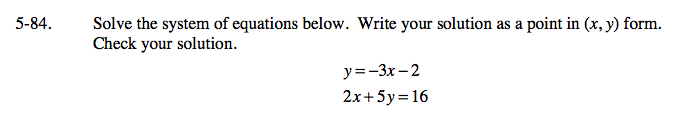### Home > GC > Chapter 5 > Lesson 5.3.2 > Problem5-84

5-84.

Solve the system of equations below. Write your solution as a point in (x, y) form. Check your solution. Homework Help ✎

y = −3x − 2
2x + 5y = 16Substitute the first equation into the second to solve for one variable.

2x + 5(−3x − 2) = 16

Once you have one of the variables, solve for the other.